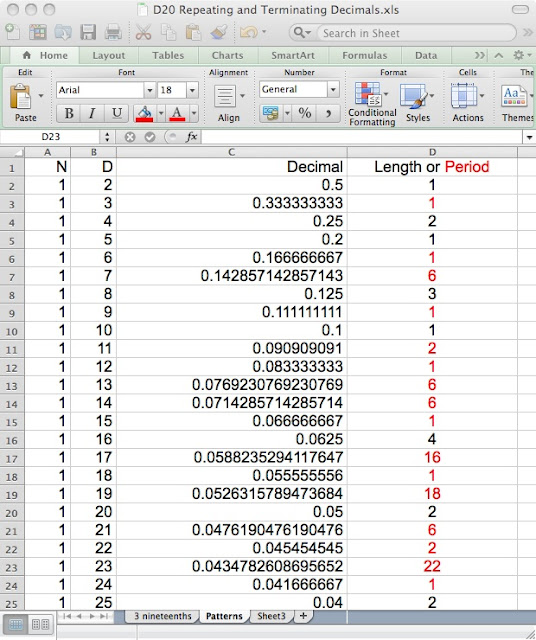## Tuesday, November 13, 2012

### When will it end?

[In the United States] Teachers act as if student interest will be generated only by diversions outside of mathematics. (The Teaching Gap, p. 89)
Schema Activation: Journal Jot
Describe the process of converting three-nineteenths to a decimal

Focus: Changing the Script
We have read in The Teaching Gap how teaching mathematics in the United States typically focuses on preparing students to perform prepackaged procedures. What if we tried to change the focus from mathematical procedures to mathematical practices?
In this workshop, try to refocus your efforts away from simply following a mindless process and explore what new knowledge is waiting to be learned. As you work on the following problem, please keep track of how you are (or aren't) engaging in these practices.

Activity: Find the exact decimal representation for three-nineteenths

[The follow represents how learners have engaged with this activity.]

This seems like a fairly straightforward exercise. Why not just plug the numbers into a calculator? The converting-a-fraction-to-a-decimal procedure requires us to divide the numerator by the denominator until the decimal either repeats or terminates. Three divided by nineteen - easy. Except, the quotient displayed on the calculator screen does not provide enough information since it does not show enough places.

Maybe using a different calculation tool would help. What about an Excel spreadsheet?
This seems to suggest that three-nineteenths terminates. And it matches the calculator's answer. A problem solver would not simply accept these and be done - would she?

What other strategies could apply?
• Work the division out by hand
• See if another related fraction might shed some light on the decimal
• 1/19 since 1/19 multiplied by 3 is 3/19
• 16/19 since this would be the complement of 3/19
• Find x such that 19x = 100 and then calculate 3x
• Multiply 0.157894736842105 by 19 to see if the product is 3
[Learners often explore these approaches with various levels of success. Having monitored their own progress, the learners often turn to other strategies or decide to seek a solution to other related problems. They do not want to be stuck trying the same approach over and over again.

WolframAlpha has added a wrinkle to this activity as it provides an exact decimal representation for three-nineteenths. Originally, we thought this would be problematic as it gives a clear answer to the original question. It is interesting, however, that focusing on a problem solving approach generates similar alternative/extension problems whether or not the answer has been found.]

New problems:
• Which fractions repeat and which terminate? Why?
• Can we predict the period of the decimal representation of a given fraction?Partial table created by learners in order to look for patterns
[Answers to these alternative/extension question rarely are answered in the time available to the workshop. Learners are encouraged to continue exploring the problems if they are interested. Even though they have not come to any firm conclusions, they usually have some interesting answers to the reflection questions.]

Reflection: Mathematician's Chair
• What did you do as it relates to the mathematical practices?
1.